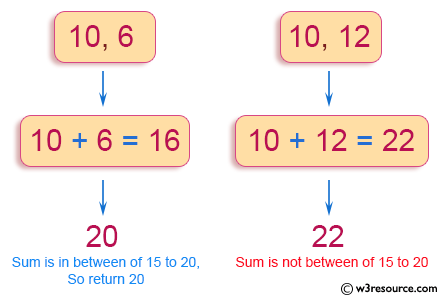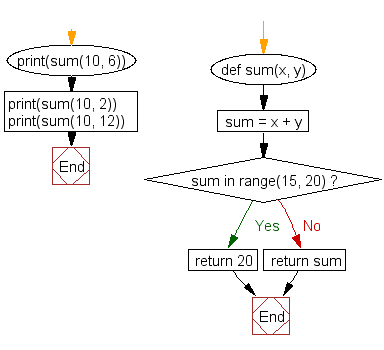﻿ Python Exercise: Sum of two given integers. However, if the sum is between 15 to 20 it will return 20 - w3resource# Python Exercise: Sum of two given integers. However, if the sum is between 15 to 20 it will return 20

## Python Basic: Exercise-34 with Solution

Write a Python program to sum of two given integers. However, if the sum is between 15 to 20 it will return 20.

Pictorial Presentation:Sample Solution:

Python Code:

``````def sum(x, y):
sum = x + y
if sum in range(15, 20):
return 20
else:
return sum

print(sum(10, 6))
print(sum(10, 2))
print(sum(10, 12))
```
```

Sample Output:

```20
12
22
```

Flowchart :## Visualize Python code execution:

The following tool visualize what the computer is doing step-by-step as it executes the said program:

Python Code Editor:

Have another way to solve this solution? Contribute your code (and comments) through Disqus.

What is the difficulty level of this exercise?

Test your Python skills with w3resource's quiz

﻿

## Python: Tips of the Day

Returns a list with n elements removed from the beginning

Example:

```def tips_take(itr, n = 1):
return itr[:n]
print(tips_take([1, 2, 3], 5))
print(tips_take([1, 2, 3], 0))
```

Output:

```[1, 2, 3]
[]
```